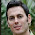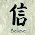## Friday, July 15, 2011

### Relationship between osmosis process and hypertonic, hypotonic and isotonic effects

Objective:

1. To understand the phenomenon in which molecules flow from a location of higher chemical potential to a region of lower potential in which both regions are separated by a semi permeable membrane.

2. To investigate the hypertonic, hypotonic and isotonic effect.

Introduction:

Osmosis is the net diffusion of water molecules from a region of lower solute concentration to a region of higher concentration by passing through a semi permeable membrane. A semi permeable membrane is otherwise known as selectively membrane which only allows some of the molecules to pass through it but not others. However, the membrane is permeable to the solvent. The permeability is depends on the size and property of molecule. In osmosis, the net movement of water molecules will only move in one direction. The water molecules move from the side where water concentration is higher to the other side of the membrane which down the concentration gradient.

Osmosis is a passive process which does not involve any energy input into the system. The osmosis process is spontaneously and naturally. The difference in the concentration of solutions creates an osmotic pressure. Osmotic pressure is the pressure that exists across a semi permeable membrane between two solutions of different concentration. Osmotic pressure of a solution is a colligative property and at given temperature its magnitude depends only on the concentration of the solute but not its identity. Osmotic pressure is calculated by using the van't Hoff's formula below:

Π = cRT

where π = osmotic pressure (atm)

c = concentration of solution (mol/L)

R = gas constant

T = Temperature(K)

The concentration of solution can be categorized into three basic categorizes which have different concentration with respect to a particular concentration of solution. The types of solution are divided into hypertonic, hypotonic, and isotonic. Hypertonic solution is the solution containing a higher solute concentration when compared to a particular solution. The higher the solute concentration, the lower the water potential and osmotic pressure. This is because some water molecules will be attracted to the solute molecule and will no longer be free. In osmosis, the water molecules will flow into the hypertonic solution due to lower water potential. For hypotonic solution, is defined as a solution with lower solute concentration with respect to a solution. Its water potential and osmotic pressure are higher. In hypotonic solution, there are more free water molecules which always diffuse into a region of lower water potential. The solution has same concentration respect to another solution is known as isotonic solution. It has the same water potential and osmotic pressure which the net movements of water molecules are the same for both solutions.

Net movement of the water molecules are from hypotonic (low-concentrated solute) to hypertonic (high-concentrated solute) due to difference of osmotic pressure in two solutions. Due to the thermal agitation, the water molecules will diffuse spontaneously (Δ G < 0) from the region of lower solute concentration towards higher solute concentration. The addition of solute will increase the entropy of water molecules since the solute is being dispersed overall in the solution. The direct osmosis (follow concentration gradient) is entropy generating process (ΔS > 0) which having tendency to equalize the chemical potentials and concentrations of two different solutions which are separated by the semi permeable membrane. The water is acting to dilute the solution with higher solute concentration until the concentration is the same for both sides. However, the water will continue to flow with the similar rate in the both directions.

Apparatus: 50ml beaker, analytical balance

Materials: dialysis tubing, tap water, 30% sucrose solution, 60% sucrose solution, string

Procedure:

1. Three pieces of equal length of dialysis tubing and several lengths of string were obtained.

2. One end of the tubing was folded over and tied closed with the string.

3. To each tube, 5 ml of 30% sucrose solution was added. Then, the bag was squeezed gently to remove excess air and was tied off with string.

4. Some slack was left in the bag as room for expansion, but the air trapped inside was removed. The bags were briefly rinsed in running tap water and then were dried on paper towels.

5. Each bag was weighed and the results were recorded.

6. After weighing, three bags were placed into tap water, 30% sucrose solution and 60% sucrose solution each.

7. The bags were remained undisturbed for 30 minutes.

8. The bags were removed, rinsed, and reweighed. All results were recorded into table.

Results and calculations:

 Tap water 30% Sucrose 60% sucrose Weight at 0 min 5.7739g 5.9171g 5.9795g 30 min 7.1150g 5.8309g 5.3015g Change 1.3411g -0.0862g -0.6780g

Percentage of weight of dialysis tubing (in tap water) increase

= 1.3411g/5.7739g x 100%

= 23.22%

Percentage of weight of dialysis tubing (in 30% sucrose solution) decrease

= 0.0862g/ 5.8309g x 100%

= 1.48%

Percentage of weight of dialysis tubing (in 60% sucrose solution) decrease

= 0.6780g/5.9795g x 100%

= 11.34%

Discussion:

In this experiment, three dialysis tubing were filled with 5 ml of 30% sucrose solution. These dialysis tubing were separately put into the three beaker which containing tap water, 30% sucrose solution and 60% sucrose solution respectively. The dialysis tubing is semi permeable which allows some molecules that are small enough to pass through it. In this case, the small molecules are referred to water molecules. The contents in the dialysis tubing are not flow out through the small pores before place them into beakers with 30% sucrose solution. This may be due to the cohesion force between the water molecules in which try to pull the outermost water molecules in dialysis tubing inwardly.

When one of the dialysis tubing containing 30% sucrose is placed into the beaker with tap water, this tap water is said to be hypotonic with respect to 30% sucrose solution. Due to the high water potential in tap water, the water molecules will tend to move from the tap water into the dialysis tubing. The osmotic pressure of tap water is higher than the osmotic pressure of sucrose solution. The water molecules are forced to move out from the tap water to 30% sucrose solution and move to 30% sucrose solution by diffusing the membrane of dialysis tubing. The reason is because the higher osmotic pressure in the tap water forced the water molecules to diffuse into sucrose solution. Eventually, the rate of water diffuse into the tubing is higher than the rate of water diffuse out from the tubing until equilibrium is reached. The increase in the water caused the weight of dialysis increases as well.

The 60% sucrose solution is considered as hypertonic solution when compared to the 30% sucrose solution in it. The 60% sucrose solution has higher solute concentration and lower water potential due to lack of free water molecules. This is because more water molecules are attracted to the sucrose molecules in the solution and hence the particular water molecules are not free to move. The low osmotic pressure and low water potential in 60% sucrose solution causes the water molecules in 30% sucrose solution to move into it by osmosis. Spontaneously, a net movement of water tends to minimize the difference in concentration between two solutions. The diffusing rate of water molecules move out from the dialysis tubing is higher than the water molecules move into the particular tubing. As a result, the volume of tubing has been decreased as well as its weight. The net movement will become zero after the same concentration of solution is reached for both sides of dialysis membrane.

Isotonic solution is a solution has the same concentration with respect to another solution. In this experiment, one of the 30% sucrose solutions was placed into a beaker containing 30% sucrose solution. So, the solution in the beaker is called isotonic since it has the same concentration with the solution in dialysis tubing. The water potentials of both solutions are the same as the two solutions have the same amount of water molecules free to move in the solutions. Thus, the rates of water molecules diffuse in and out of dialysis tubing are the same since there is no difference in osmotic pressure for both solutions. The net movement of water molecules is zero as the water potentials between two solutions has reached equilibrium. Theoretically, the weight and volume of dialysis has no change. However, the weight of dialysis tubing was reduced in practical. This might be due to the content in beaker was contaminated by impurity which caused the water molecules to move out. The second reason might be due to there is some water left inside the tubing which diluted its content, so the concentration has been reduced and the solution is beaker is considered as hypertonic with respect to the sucrose solution in dialysis tubing. The decrease in concentration might be small but it is enough to dilute the contents which caused the water potential increased and hence diffuse out to the solution (hypertonic) in the beaker.

Precaution steps:

1. The dialysis tubing should not be too tight and turgid in order to leave some slack in the tubing as space for expansion when water flow into it.

2. The exterior of dialysis tubing should be roughly dried before weighing to prevent the weight of water is being counted.

3. The interior of dialysis tubing should be dried in order to prevent dilution of sucrose solution.

1.2.